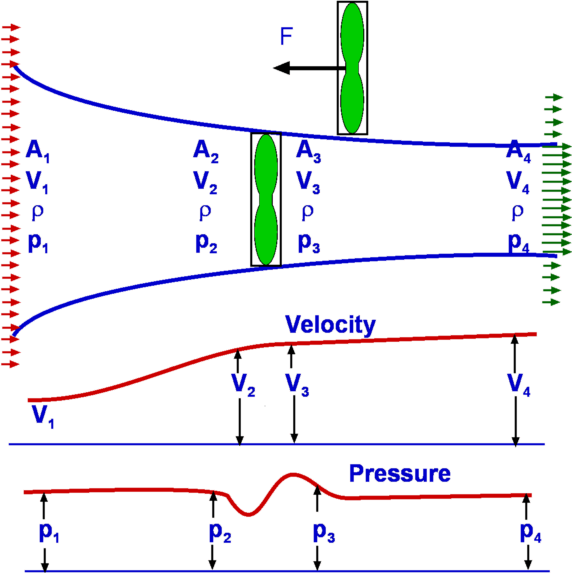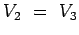## Froude's Propeller Theory

Propellers are a mechanism for the propulsion of an aeroplane. In its generic form a propeller is pair of rotating blades mounted on a shaft that houses the engine as well. As the engine operates the propeller turns sucking a large amount of air. As this air passes through the rotating blades, it gets energised, its speed increases. In the process the required Thrust to propel the aircraft is produced.Figure 3.31: Froude analysis of a Propeller

The analysis we carry out follows William Froude (1810-1879). We consider the propeller as a thin disc rotating in air as shown in Fig.3.31. Let the pressure and velocity far away from the disc i.e., at section (1) be p1 and V1 respectively. The conditions just at (2) which is the front of the disc are p2 and V2. The disc imparts momentum and energy to the incoming air such that the pressure and velocity just behind the disc (3) are V3 and p3 respectively. At (4), far downstream the conditions are V4 and p4.

We assume that the air which is influenced by the disc is confined to a slipstream as shown.

Since the disc is thin and the area of cross section at (2) and (3) ar equal, we have(3.100)

The pressures at (1) and (4) are equal to the freestream value.

We consider the control volume formed by slipstream and the ends (1) and (4) and write the momentum equation.

Subsections (c) Aerospace, Mechanical & Mechatronic Engg. 2005
University of Sydney